Home Practice
For learners and parents For teachers and schools
Textbooks
Full catalogue
Pricing SupportLog in

We think you are located in United States. Is this correct?

# 8.5 Similarity

## 8.5 Similarity (EMCJD)

temp text

### Similar polygons (EMCJF)

Polygons are similar if they are the same shape but differ in size. In other words, one polygon is an enlargement of the other. Congruent polygons are also similar since they are the same shape and the same size, only their position or orientation is different.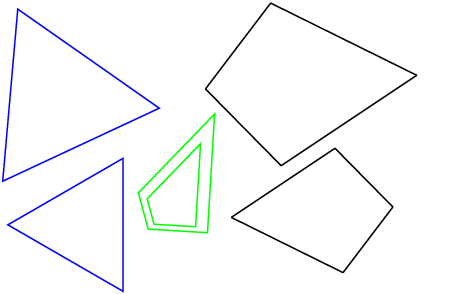Two polygons with the same number of sides are similar when:

1. All pairs of corresponding angles are equal, and
2. All pairs of corresponding sides are in the same proportion.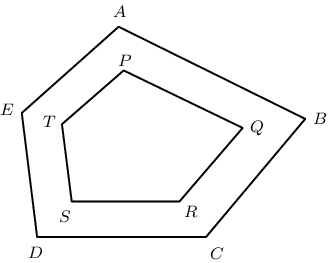In the diagram above, polygon $$ABCDE$$ is similar to polygon $$PQRST$$ if:

Condition 1:

$\hat{A} =\hat{P}, \quad \hat{B} =\hat{Q}, \quad \hat{C} =\hat{R}, \quad \hat{D} =\hat{S}, \quad \hat{E} =\hat{T}$

AND

Condition 2:

$\frac{AB}{PQ}=\frac{BC}{QR}=\frac{CD}{RS}=\frac{DE}{ST}=\frac{EA}{TP}$

Important:

• Both conditions must be true for two polygons to be similar.
• If we are given two polygons that are similar, then we know that both conditions are true.

## Worked example 4: Similar polygons

Polygons $$PQTU$$ and $$PRSU$$ are similar. Determine the value of $$x$$.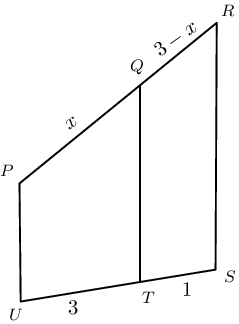### Identify pairs of corresponding sides

Since the two polygons are similar,

\begin{align*} \frac{PQ}{PR} & = \frac{TU}{SU} \\ \therefore \frac{x}{x+\left(3-x\right)} & = \frac{3}{3 + 1} \\ \therefore \frac{x}{3} & = \frac{3}{4} \\ \therefore x & = \frac{9}{4} \end{align*}

## Similar polygons

Textbook Exercise 8.6

Determine whether or not the following polygons are similar, giving reasons.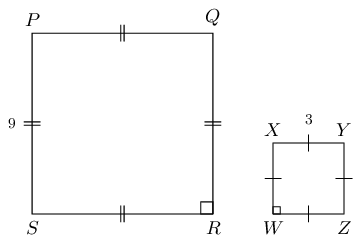Similar, all angles $$= \text{90}°$$ and sides are in the same proportion.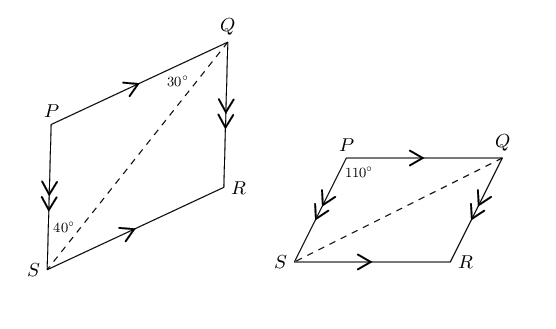Not enough information is given. Corresponding angles are equal.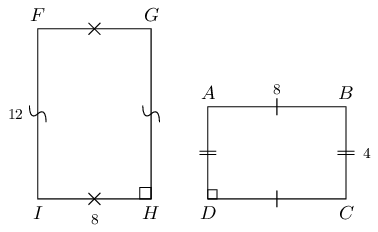Not similar, sides are not in the same proportion.

Are the following statements true or false? If false, state reasons or draw an appropriate diagram.

All squares are similar.
True
All rectangles are similar.

False. Pairs of corresponding sides not necessarily in the same proportion.

All rhombi are similar.

False. Corresponding angles not necessarily equal.

All congruent polygons are similar.
True
All similar polygons are congruent.

False. Corresponding angles are equal but not necessarily true that corresponding sides are equal.

All congruent triangles are similar.
True
Isosceles triangles are similar.

False. Corresponding angles not necessarily equal and pairs of corresponding sides not necessarily in the same proportion.

Equilateral triangles are similar.
True

### Similarity of triangles (EMCJG)

To prove two polygons are similar, we need to show that two conditions are true: (a) all pairs of corresponding angles are equal and (b) all pairs of corresponding sides are in the same proportion.

To prove two triangles are similar, we need only show that one of the conditions is true. If one of the conditions is true for two triangles, then the other condition is also true.

# Chapter 8: Theorem: Equiangular triangles are similar

Equiangular triangles are similar.

(Reason: equiangular $$\triangle$$s)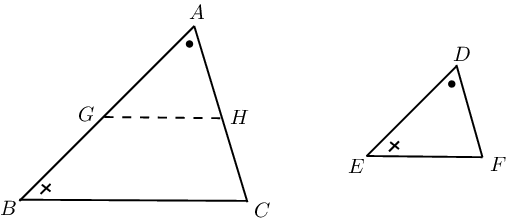$$\triangle ABC$$ and $$\triangle DEF$$ with $$\hat{A}=\hat{D}$$; $$\hat{B}=\hat{E}$$; $$\hat{C}=\hat{F}$$

$$\frac{AB}{DE}=\frac{AC}{DF} = \frac{BC}{EF}$$

Construction: mark $$G$$ on $$AB$$ so that $$AG=DE$$, and mark $$H$$ on $$AC$$ so that $$AH=DF$$.

In $$\triangle AGH$$ and $$\triangle DEF$$

$\begin{array}{rll} AG&=DE & \text{(by construction)} \\ AH&=DF& \text{(by construction)} \\ \hat{A}&=\hat{D} &\text{(given)} \\ \triangle AGH &\equiv \triangle DEF &\text{(SAS)} \\ \therefore A\hat{G}H &=\hat{E} & \\ \hat{E} &= \hat{B} & \text{(given)} \\ \therefore A\hat{G}H &=\hat{B} & \\ \therefore GH &\parallel BC &\text{(corresp. }\angle \text{s}\text{ equal)} \end{array}$ $\begin{array}{rll} \frac{AB}{AG}&=\frac{AC}{AH} &\text{(prop. }\text{theorem)} \\ \therefore \frac{AB}{DE}&=\frac{AC}{DF} &\text{(}AG=DE\text{, }AH=DF\text{)} \end{array}$

Similarly, by constructing $$Q$$ on $$CA$$ so that $$CQ = FD$$, and mark $$P$$ on $$BC$$ so that $$CP=FE$$.

$\begin{array}{rll} \frac{CA}{FD} &= \frac{CB}{FE} & \\ & & \\ \therefore \frac{AB}{DE}&=\frac{AC}{DF} = \frac{BC}{EF} & \\ & & \\ \therefore \triangle ABC & \enspace ||| \enspace \triangle DEF & \end{array}$

Therefore equiangular triangles are similar.

Notation

• The symbol for congruency is $$\equiv$$.

• The symbol for similarity is $$|||$$.

• Be careful to label similar triangles correctly.

For example, if $$\hat{P} = \hat{B}$$, $$\hat{Q} = \hat{A}$$ and $$\hat{R} = \hat{C}$$, then $$\triangle PQR \enspace ||| \enspace \triangle BAC$$.

Do not write $$\triangle PQR \enspace ||| \enspace \triangle ABC$$.

• $$\triangle PQR \enspace ||| \enspace \triangle BAC$$ also indicates which sides of the triangles are in the same proportion:

$$\frac{PQ}{BA} = \frac{QR}{AC} = \frac{PR}{BC}$$

temp text

Proving equiangular triangles are similar:

The sum of the interior angles of any triangle is $$\text{180}$$°. If we know that two pairs of angles are equal, then the remaining angle in each triangle must also be equal. Therefore the two triangles are similar.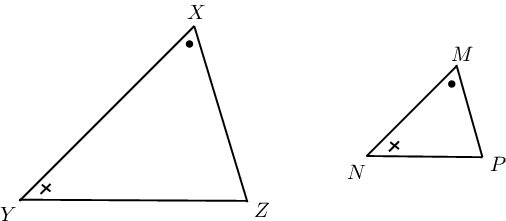In other words, to prove that two triangles are equiangular, we need only show that two pairs of angles are equal.

## Worked example 5: Similarity of triangles

Prove that $$\triangle XYZ$$ is similar to $$\triangle SRT$$.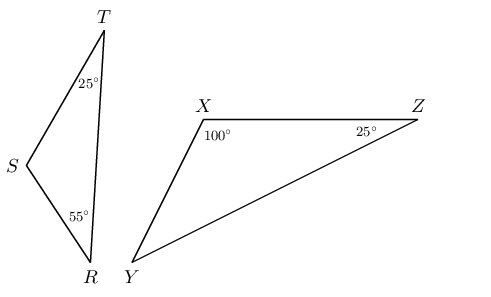### Calculate the unknown angles in each triangle

$\begin{array}{rll} \text{In } \triangle XYZ: \quad \hat{Y} &= \text{180}° - \left( \text{180}° + \text{25}° \right) & (\text{sum of int. } \angle \text{s of } \triangle) \\ &= \text{55}° & \\ & & \\ & & \\ \text{In } \triangle SRT: \quad \hat{S} &= \text{180}° - \left( \text{55}° + \text{25}° \right) & (\text{sum of int. } \angle \text{s of } \triangle) \\ &= \text{100}° & \end{array}$

### Prove that the two triangles are similar

$\begin{array}{rll} \text{In } \triangle XYZ & \text{and } \triangle SRT: & \\ \hat{X} &= \hat{S} = \text{100}° & (\text{proved}) \\ \hat{Z} &= \hat{T} = \text{25}° & (\text{given}) \\ \therefore \triangle XYZ \enspace & ||| \enspace \triangle SRT & (\text{AAA}) \end{array}$

## Similarity of triangles

Textbook Exercise 8.7

In the diagram below, $$AB \parallel DE$$.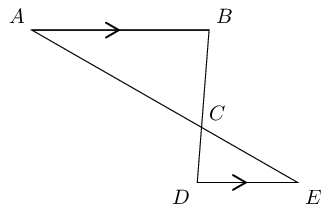Prove $$\triangle ABC \enspace ||| \enspace \triangle EDC$$.

In $$\triangle ABC \text{ and } \triangle EDC:$$

$\begin{array}{rll} \hat{A}&= \hat{E} & (\text{alt. } \angle \text{s, } AB \parallel DE ) \\ \hat{B}&= \hat{D} & (\text{alt. } \angle \text{s, } AB \parallel DE ) \\ \therefore \triangle ABC & \enspace ||| \enspace \triangle EDC & (\text{AAA}) \end{array}$

If $$\dfrac{AC}{AE} = \dfrac{5}{7} \text{ and } AB = \text{4}\text{ cm}$$, calculate the length of $$DE$$ (correct to one decimal place).

In $$\triangle ABC \text{ and } \triangle EDC:$$

$\begin{array}{rll} \dfrac{AC}{AE}&= \dfrac{5}{7} & (\text{given}) \\ & & \\ \therefore \dfrac{AC}{CE} &= \dfrac{5}{2} & \\ & & \\ \dfrac{DE}{AB} &= \dfrac{CE}{AC} & (\triangle ABC \enspace ||| \enspace \triangle EDC) \\ & & \\ \dfrac{DE}{4} &= \dfrac{2}{5} & \\ & & \\ \therefore DE &= \dfrac{8}{5} & \\ & & \\ &= \text{1,6}\text{ cm} & \end{array}$

In circle $$O$$, $$RP \perp PQ.$$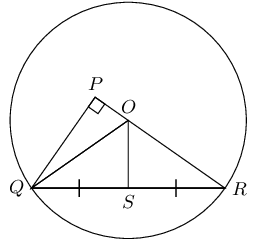Prove $$\triangle PRQ \enspace ||| \enspace \triangle SRO$$.

In $$\triangle PRQ \text{ and } \triangle SRO$$:

$\begin{array}{rll} \hat{P} &= \text{90}° & (\text{given}) \\ \hat{S} &= \text{90}° & (QS = SR) \\ \therefore \hat{P} &= \hat{S} & \\ \hat{R} &= \hat{R} & (\text{common } \angle) \\ \therefore \triangle PRQ & \enspace ||| \enspace \triangle SRO & (\text{AAA}) \end{array}$

Prove $$\dfrac{OR}{SR} = \dfrac{QR}{PR}$$.

$\begin{array}{rll} \dfrac{PR}{SR}&= \dfrac{RQ}{RO} = \dfrac{PQ}{SO} & (\triangle SRO \enspace ||| \enspace \triangle PRQ) \\ & & \\ \text{So } \quad \dfrac{RQ}{RO} & = \dfrac{PR}{SR} & \\ & & \\ \dfrac{QR}{OR} & = \dfrac{PR}{SR} & \\ & & \\ QR \cdot SR & = PR \cdot OR & \\ & & \\ \therefore \dfrac{QR}{PR} &= \dfrac{OR}{SR} & \end{array}$

If $$SR = \text{18}\text{ mm} \text{ and } QP = \text{20}\text{ mm}$$, calculate the radius of circle $$O$$ (correct to one decimal place).

$\begin{array}{rll} \dfrac{OR}{SR}&= \dfrac{QR}{PR} & (\text{proved}) \\ & & \\ \text{In } \triangle PQR: \quad PR^{2} &= QR^{2} - QP^{2} & (\text{Pythagoras}) \\ &= (36)^2 - (20)^2 & \\ \therefore PR &= \sqrt{896} & \\ &= 8\sqrt{14} & \\ \therefore \dfrac{OR}{18} &= \dfrac{36}{8\sqrt{14}} & \\ \therefore OR &= 18 \times \dfrac{36}{8\sqrt{14}} & \\ \therefore \text{radius} &= \text{21,6}\text{ mm} & \end{array}$

Given the following figure with the following lengths, find $$AE$$, $$EC$$ and $$BE$$.

$$BC = \text{15}\text{ cm}, AB = \text{4}\text{ cm}, CD = \text{18}\text{ cm} \text{ and } ED = \text{9}\text{ cm}.$$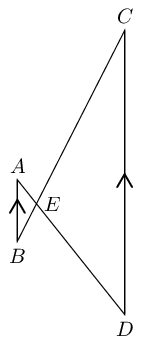$\begin{array}{rll} B\hat{A}E &=C\hat{D}E & (\text{alt. } \angle \text{s, } AB \parallel CD) \\ A\hat{B}E &=D\hat{C}E & (\text{alt. } \angle \text{s, } AB \parallel CD) \\ A\hat{E}B &=D\hat{E}C & (\text{vert. opp. } \angle \text{s}) \\ \therefore \triangle AEB & \enspace ||| \enspace \triangle DEC & (\text{AAA}) \\ & & \\ \therefore \dfrac{AE}{DE} &= \dfrac{AB}{DC} = \dfrac{4}{18} = \dfrac{2}{9} & (\triangle AEB \enspace ||| \enspace \triangle DEC) \\ & & \\ AE &= \frac{2}{9} DE & \\ & & \\ &= \frac{2}{9} (9) & \\ & & \\ &= \text{2}\text{ cm} & \end{array}$ $\begin{array}{rll} \dfrac{EC}{BC} &= \dfrac{ED}{AD} = \dfrac{9}{11} & (AB \parallel CD) \\ & & \\ EC &= \dfrac{ED}{AD} (BC) & \\ & & \\ &= \frac{9}{11} (15) & \\ & & \\ &= \text{12,3}\text{ cm} & \end{array}$ $\begin{array}{rll} \dfrac{BE}{BC} &= \dfrac{AE}{AD} = \dfrac{2}{11} & (AB \parallel CD) \\ & & \\ BE &= \dfrac{AE}{AD} (BC) & \\ & & \\ &= \frac{2}{11} (15) & \\ & & \\ &= \text{2,7}\text{ cm} & \end{array}$

# Theorem: Triangles with sides in proportion are similar

If the corresponding sides of two triangles are in proportion, then the two triangles are similar.

(Reason: sides of $$\triangle$$s in prop.)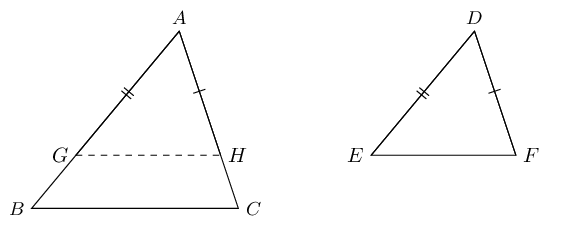$$\triangle ABC$$ and $$\triangle DEF$$ with $$\frac{AB}{DE}=\frac{AC}{DF}=\frac{BC}{EF}$$
$$\hat{A}=\hat{D}$$, $$\hat{B}=\hat{E}$$ and $$\hat{C}=\hat{F}$$

Construction: draw $$GH$$ such that $$AG=DE$$ and $$AH=DF$$.

$\begin{array}{rll} \dfrac{AB}{DE} &= \dfrac{AC}{DF} &(\text{given}) \\ & \\ \dfrac{AB}{AG} &= \dfrac{AC}{AH} &(\text{by construction}) \\ & \\ \therefore GH &\parallel BC &(\text{sides in prop.}) \\ & \\ \therefore \hat{B} &= A\hat{G}H &(\text{corresp. } \angle \text{s, }GH \parallel BC) \\ & \\ \text{and } \hat{C} &= A\hat{H}G &(\text{corresp. } \angle \text{s, }GH \parallel BC) \\ & \\ \therefore \triangle AGH & \enspace ||| \enspace \triangle ABC & ( \text{equiangular } \triangle \text{s})\\ & \\ \therefore \dfrac{GH}{BC} &= \dfrac{AG}{AB} & \\ & \\ &= \dfrac{DE}{AB} &(AG=DE \text{, by construction}) \\ & \\ &= \dfrac{EF}{BC} & \left(\text{given } \frac{AB}{DE}=\frac{BC}{EF} \right) \\ & \\ \therefore GH &=EF &\\ & \\ \therefore \triangle AGH &\equiv \triangle DEF & (\text{SSS}) \\ & \\ \therefore \triangle ABC &\text{ and } \triangle DEF \text{ are equiangular} &\\ & \\ \therefore \triangle ABC & \enspace ||| \enspace \triangle DEF & \\ \end{array}$

# Theorem: Triangles with two sides in proportion and equal included angles, are similar (PROOF NOT FOR EXAMS)

If two sides of one triangle are in proportion to two sides of another triangle and the included angles are equal, then the two triangles are similar.

(Reason: $$\triangle$$s with $$\text{2}$$ sides in prop. and equal incl. $$\angle$$s)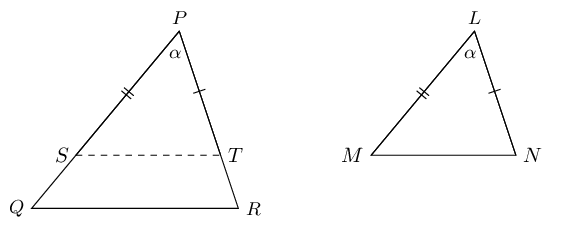$$\triangle PQR$$ and $$\triangle LMN$$ with $$\dfrac{LM}{PQ}=\dfrac{LN}{PR}$$ and $$\hat{L}=\hat{P} = \alpha$$
$$\hat{M}=\hat{Q}$$ and $$\hat{N}=\hat{R}$$

Construction: draw $$ST$$ such that $$PS=LM$$ and $$PT=LN$$.

In $$\triangle PQR$$ and $$\triangle LMN$$: $\begin{array}{rll} PS&=LM &(\text{by construction}) \\ & \\ \hat{P}&= \hat{L} & (\text{given}) \\ & \\ PT&= LN & (\text{by construction}) \\ & \\ \therefore \triangle PST & \equiv \triangle LMN &(\text{SAS}) \\ & \\ \therefore P\hat{S}T &=\hat{M} &\\ & \\ P\hat{T}S &=\hat{N} &\\ & \\ \text{and } \dfrac{PS}{PQ} &= \dfrac{PT}{PR} &(\text{by construction}) \\ & \\ \therefore ST &\parallel QR &(\text{sides in prop.}) \\ & \\ \therefore \hat{Q} &= P\hat{S}T=\hat{M} &(\text{corresp. } \angle \text{s, } ST \parallel QR) \\ & \\ \text{and } \hat{R} &= P\hat{T}S=\hat{N} &(\text{corresp. } \angle \text{s, } ST \parallel QR) \\ & \\ \therefore \triangle PQR &\enspace ||| \enspace \triangle LMN & (\text{equiangular } \triangle \text{s}) \end{array}$

temp text

Proving triangles with sides in proportion are similar:

To prove that the sides of two triangles are in the same proportion, we must show that all three pairs of corresponding sides are in the same proportion.

## Worked example 6: Similarity of triangles

1. In the diagram below, is $$\triangle ABC \enspace ||| \enspace \triangle ZYX$$?

Show calculations.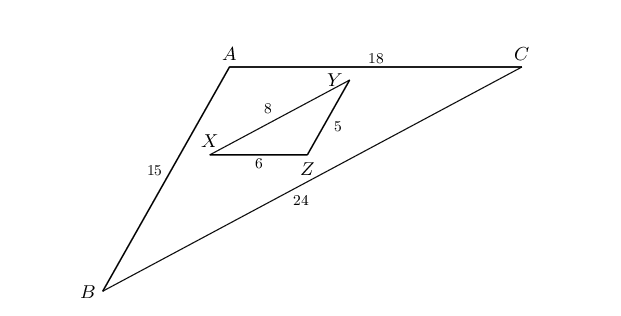2. Consider the diagram given below. Determine whether or not $$\triangle PQR \enspace ||| \enspace \triangle SPR$$.

Show calculations.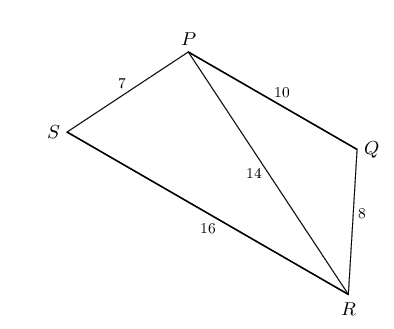(Diagrams are not drawn to scale)

### Investigate proportionality of $$\triangle ABC$$ and $$\triangle ZYX$$

For similar triangles, the order in which the triangles are labelled indicates which pairs of sides correspond.

\begin{align*} \text{In } \triangle ABC &\text{ and } \triangle ZYX: \\ \frac{AB}{ZY} &= \frac{15}{5} = \frac{3}{1} \\ \frac{AC}{ZX} &= \frac{18}{6} = \frac{3}{1} \\ \frac{BC}{YX} &= \frac{24}{8} = \frac{3}{1} \\ \therefore \triangle ABC \enspace & ||| \enspace \triangle ZYX \qquad \text{(SSS)} \end{align*}

### Investigate proportionality of $$\triangle PQR$$ and $$\triangle RPS$$

\begin{align*} \text{In } \triangle PQR &\text{ and } \triangle RPS: \\ \frac{PQ}{RP} &= \frac{10}{14} = \frac{5}{7} \\ \frac{PR}{RS} &= \frac{14}{16} = \frac{7}{8} \\ \frac{QR}{PS} &= \frac{8}{7} \\ \therefore \triangle PQR &\text{ is not similar to } \triangle RPS \end{align*}

## Worked example 7: Similarity of triangles

$$PQSR$$ is a trapezium, with $$PQ \parallel RS$$. Prove that $$PT \cdot RT = ST \cdot QT$$.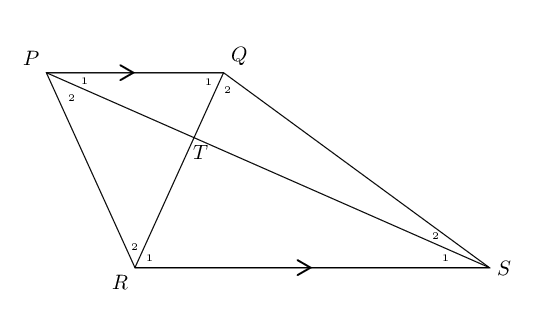### Identify triangles

We are required to prove that $$PT \cdot RT = ST \cdot QT$$, which we can also write as the ratio $$\frac{PT}{QT} = \frac{ST}{RT}$$.

To determine which two triangles we must consider, examine the lengths given in the ratio.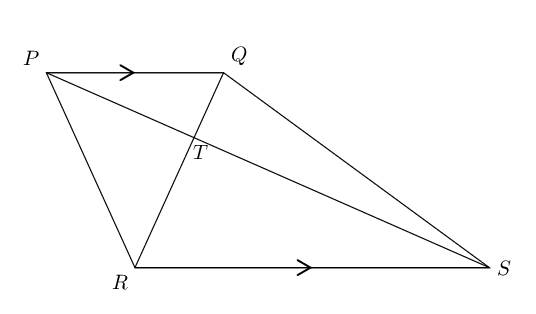### Prove triangles are equiangular

$\begin{array}{rll} \text{In } \triangle PTQ \text{ and } \triangle STR: \quad \hat{{P}_{1}} & = \hat{{S}_{1}} & (\text{alt. }\angle \text{s}, PQ \parallel RS) \\ \hat{{Q}_{1}} & = \hat{{R}_{1}} & (\text{alt. } \angle \text{s}, PQ \parallel RS) \\ \therefore \triangle PTQ & \enspace ||| \enspace \triangle STR & \text{(AAA)} \end{array}$

### Use proportionality

$\begin{array}{rll} \frac{PT}{TQ} & = \frac{ST}{TR} & \text{(}\triangle PTQ \enspace ||| \enspace \triangle STR\text{)} \\ \therefore PT \cdot TR & = ST \cdot TQ & \end{array}$

## Similarity of triangles

Textbook Exercise 8.8

Consider the diagram given below. $$PR = 20$$ units and $$XZ = 12$$ units. Is $$\triangle XYZ \enspace ||| \enspace \triangle PQR$$? Give reasons.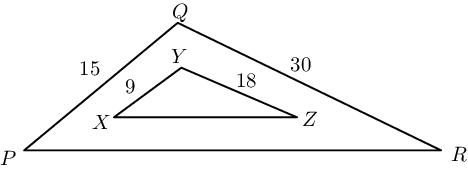\begin{align*} \frac{XY}{PQ} &= \frac{9}{15} = \frac{3}{5} \\ \frac{YZ}{QR} &= \frac{18}{30} = \frac{3}{5} \\ \frac{ZX}{RP} &= \frac{12}{20} = \frac{3}{5} \end{align*}

Yes, $$\triangle XYZ \enspace ||| \enspace \triangle PQR$$.

$$AB$$ is a diameter of the circle $$ABCD$$. $$OD$$ is drawn parallel to $$BC$$ and meets $$AC$$ in $$E$$.

If the radius is $$\text{10}$$ $$\text{cm}$$ and $$AC = \text{16}\text{ cm}$$, calculate the length of $$ED$$.

[NCS, Paper 3, November 2011]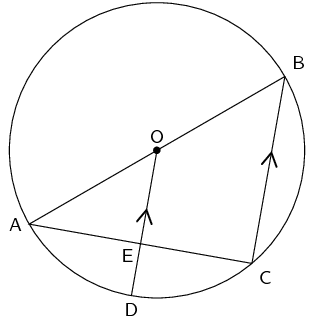$\begin{array}{r@{\;}l@{\quad}l} \hat{C} & = \text{90}° & (\angle \text{s in semi-circle})\\ O\hat{E}A & = \text{90}° & (\text{corresp. } \angle \text{s; } OD \parallel BC)\\ AE & = \text{8}\text{ cm} & (\text{line from centre } \perp \text{chord})\\ OE & = \text{6}\text{ cm} & (\text{Pythagoras})\\ ED & = \text{10} - \text{6} = \text{4}\text{ cm} & \end{array}$

OR

$\begin{array}{r@{\;}l@{\quad}l} \hat{C} & = \text{90}° & (\angle \text{s in semi-circle})\\ O\hat{E}A& = \text{90}° & (\text{corresp. } \angle \text{s; } OD \parallel BC)\\ OE & \parallel BC & (\text{given})\\ OA & = OB & (\text{radii})\\ AE & = EC = \text{8}\text{ cm} & (\text{midpoint th.})\\ OE &= \text{6}\text{ cm} & (\text{Pythagoras})\\ ED &= \text{10} - \text{6} = \text{4}\text{ cm} & \end{array}$

OR

$\begin{array}{r@{\;}l@{\quad}l} \hat{C} &= \text{90}° & (\angle \text{s in semi-circle})\\ BC^{2} &= (20)^{2} - (16)^{2} & \\ BC^{2} &= 144 &\\ BC &= 12 &\\ OE &= \frac{1}{2}BC & (\text{midpoint th.})\\ OE &= \text{6}\text{ cm} &\\ OD & = \text{10}\text{ cm} &\\ ED & = \text{10} - \text{6} = \text{4}\text{ cm} & \end{array}$

$$P, Q, S \text{ and } T$$ are on the circumference of the circle.

$$TS \text{ is produced to } V \text{ so that } SV = 2TS.$$

$$TRQ \text{ is produced to } U \text{ so that } VU \parallel SRP.$$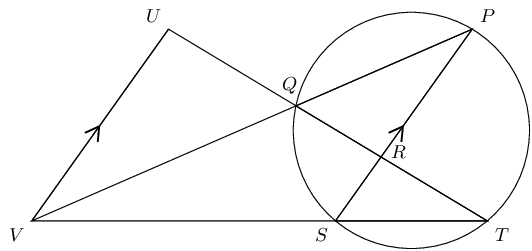Prove, with reasons, that:

$$\dfrac{TR}{RU} = \dfrac{1}{3}$$

$\begin{array}{rll} \dfrac{TR}{TU}&= \dfrac{TS}{TV} & (SR \parallel VU) \\ &= \dfrac{1}{3} & (SV = 2TS) \end{array}$

$$\triangle TQV \enspace ||| \enspace \triangle PSV$$

In $$\triangle TQV \text{ and } \triangle PSV$$:

$\begin{array}{rll} \hat{P}&= \hat{T} & (\angle \text{s subtended chord } QS)\\ P \hat{V} T&= P \hat{V}T & (\text{common }\angle) \\ \therefore \triangle TQV & \enspace ||| \enspace \triangle PSV & (\text{AAA}) \end{array}$

$$QV \cdot PV = 6TS^{2}$$

$\begin{array}{rll} \dfrac{QV}{TV}&= \dfrac{SV}{PV} & (\triangle TQV \enspace ||| \enspace \triangle PSV) \\ \therefore QV \cdot PV&= TV.SV & \\ \text{But } SV &= 2TS & (\text{given})\\ \therefore TV &= 3TS & \\ \therefore QV.PV &= 3TS.2TS & \\ QV.PV &= 6TS^{2} & \end{array}$

$$\triangle UQV \enspace ||| \enspace \triangle RQP \enspace ||| \enspace \triangle RST$$

In $$\triangle UQV \text{ and } \triangle RQP \text{ and } \triangle RST$$:

$\begin{array}{rll} \hat{U}&= Q\hat{R}P & (\text{alt. } \angle \text{s, } VU \parallel RP) \\ &= S\hat{R}T & (\text{vert. opp. } \angle \text{s}) \\ U\hat{V}Q&= \hat{P} & (\text{alt. } \angle \text{s, } VU \parallel RP) \\ &= \hat{T} & (\angle \text{s on chord } QS) \\ \triangle UQV & \enspace ||| \enspace \triangle RQP \enspace ||| \enspace \triangle RST & (\text{AAA}) \end{array}$

$$ABCD$$ is a parallelogram with diagonals intersecting at $$F$$. $$FE$$ is drawn parallel to $$CD$$. $$AC$$ is produced to $$P$$ such that $$PC = 2AC$$ and $$AD$$ is produced to $$Q$$ such that $$DQ = 2AD$$.

[NCS, Paper 3, November 2011]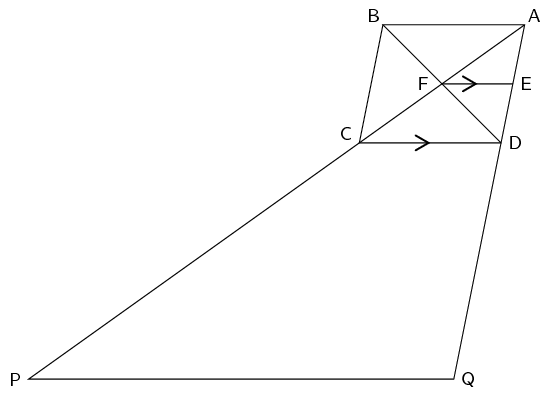Show that $$E$$ is the midpoint of $$AD$$.

$\begin{array}{r@{\;}l@{\quad}l} AF & = FC & (\text{diag. parm. bisect})\\ FE & \parallel \; CD & \\ AE & = ED & (\text{prop th. }FE \parallel CD)\\ \end{array}$

Prove $$PQ \parallel FE$$.

$\begin{array}{r@{\;}l@{\quad}l} \frac{AC}{CP} & = \frac{1}{2} & (\text{given})\\ \frac{AD}{DQ} & = \frac{1}{2} & (\text{given})\\ \frac{AC}{CP} & = \frac{AD}{DQ} &\\ CD & \parallel PQ & (\text{converse prop. th.})\\ CD & \parallel FE & (\text{given})\\ \therefore PQ & \parallel FE & \end{array}$

OR

$\begin{array}{r@{\;}l@{\quad}l} \frac{AC}{AP} & = \frac{1}{3} &\\ \frac{AD}{AQ} & = \frac{1}{3} &\\ \frac{AC}{AP} & = \frac{AD}{AQ} &\\ CD & \parallel PQ & (\text{converse prop. th.})\\ CD & \parallel FE & (\text{given})\\ \therefore PQ & \parallel FE & \end{array}$

OR

\begin{align*} \frac{AF}{AP} & = \frac{1}{6}\\ \frac{AE}{AQ} & = \frac{1}{6}\\ \frac{AF}{AP} & = \frac{AE}{AQ}\\ \therefore PQ & \parallel FE \quad(\text{converse prop. theorem}) \end{align*}

If $$PQ$$ is $$\text{60}$$ $$\text{cm}$$, calculate the length of $$FE$$.

In $$\triangle AEF$$ and $$\triangle APQ$$:

1. $$\hat{A} \quad (\text{ is common})$$
2. $$A\hat{E}F = A\hat{Q}P \quad (\text{corresp. } \angle \text{s, } FE \parallel PQ)$$
3. $$A\hat{F}E = A\hat{P}Q \quad (\text{corresp. } \angle \text{s, } FE \parallel PQ)$$

$$\therefore \triangle BHD \enspace ||| \enspace \triangle FED \quad (\angle \angle \angle)$$

\begin{align*} \frac{FE}{PQ} &= \frac{AF}{AP} \quad (||| \enspace \triangle\text{s}) \\ \frac{FE}{60} &= \frac{1}{6} \\ FE &= \text{10}\text{ cm} \end{align*}

OR

In $$\triangle ADC$$ and $$\triangle APQ$$:

1. $$\hat{A} \quad (\text{ is common})$$
2. $$A\hat{D}C = A\hat{Q}P \quad (\text{corresp. } \angle \text{s, } CD \parallel PQ)$$
3. $$A\hat{C}D = A\hat{P}Q \quad (\text{corresp. } \angle \text{s, } CD \parallel PQ)$$

$$\therefore \triangle ADC \enspace ||| \enspace \triangle APQ \quad (\angle \angle \angle)$$

$\begin{array}{r@{\;}l@{\quad}l} \frac{AC}{AP} &= \frac{AD}{AQ} = \frac{1}{3} & (||| \triangle\text{s}) \\ CD & = \frac{1}{3}PQ & \\ CD & = \text{20}\text{ cm} & \\ \text{But } AF & = FC &\\ AE & = ED & (\text{mid-point th.}) \\ FE & = \frac{1}{2}CD & \\ FE & = \text{20}\text{ cm} & \end{array}$